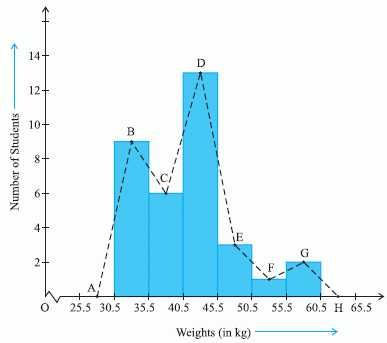Email us to get an instant 20% discount on highly effective K-12 Math & English kwizNET Programs!

#### Online Quiz (WorksheetABCD)

Questions Per Quiz = 2 4 6 8 10

### Middle/High School Algebra, Geometry, and Statistics (AGS)9.16 Frequency Polygon

 Frequency Polygons A frequency polygon is obtained by joining the middle points of the upper sides of the adjacent rectangles of the histogram by means of line segments. To complete the polygon we assume a class interval with zero frequency preceding the first class interval and a class interval with zero frequency succeeding the last class interval.Directions: On the basis of the given information answer the following questions
 Q 1: The area covered by the frequency polygon and the histogram is the same.YesNo Q 2: The class mark of the interval 30.5 - 35.5 isBACH Q 3: The class interval with maximum frequency is 35.5 - 40.545.5 - 30.530.5 - 35.540.5 - 45.5 Q 4: The class mark of the interval 40.5 - 45.5 isACBD Question 5: This question is available to subscribers only! Question 6: This question is available to subscribers only!